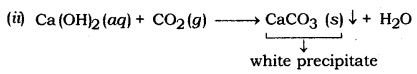# Write chemical equations for the reactions taking place when: (i) Zinc sulphide is heated in air

Write chemical equations for the reactions taking place when:
(i) Zinc sulphide is heated in air.
(ii) Carbon dioxide gas is passed into lime water.
(iii) Hydrogen sulphide is passed into an aqueous solution of SO2.

Chemical Reaction:
(i) 2Zns + 3O2(g) = 2 ZnO(s) + 2SO2(g)(iii) 2H2S + SO2 → 2H2O + 3S